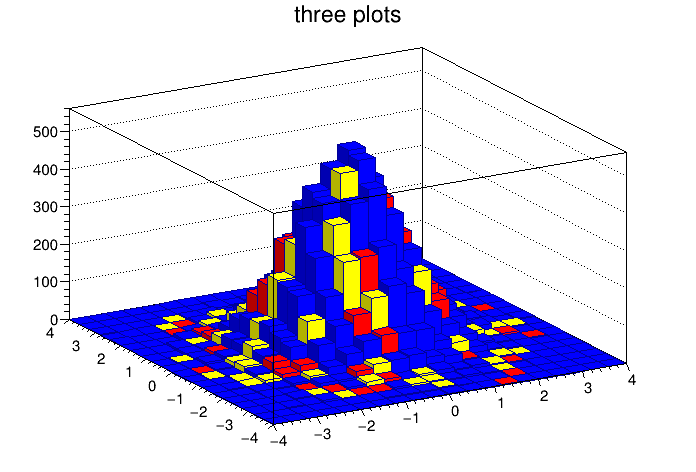# Multicolor¶

Use a THStack to show a 2-D hist with cells with different colors.

root > .x multicolor.C
root > .x multicolor.C(1)


Author: Rene Brun
This notebook tutorial was automatically generated with ROOTBOOK-izer from the macro found in the ROOT repository on Thursday, May 19, 2022 at 08:13 AM.

In :
%%cpp -d
#include "TCanvas.h"
#include "TH2.h"
#include "THStack.h"
#include "TRandom.h"


Arguments are defined.

In :
Int_t isStack=0;

In :
TCanvas *c1 = new TCanvas;
Int_t nbins = 20;
TH2F *h1 = new TH2F("h1","h1",nbins,-4,4,nbins,-4,4);
h1->SetFillColor(kBlue);
TH2F *h2 = new TH2F("h2","h2",nbins,-4,4,nbins,-4,4);
h2->SetFillColor(kRed);
TH2F *h3 = new TH2F("h3","h3",nbins,-4,4,nbins,-4,4);
h3->SetFillColor(kYellow);
THStack *hs = new THStack("hs","three plots");
TRandom r;
Int_t i;
for (i=0;i<20000;i++) h1->Fill(r.Gaus(),r.Gaus());
for (i=0;i<200;i++) {
Int_t ix = (Int_t)r.Uniform(0,nbins);
Int_t iy = (Int_t)r.Uniform(0,nbins);
Int_t bin = h1->GetBin(ix,iy);
Double_t val = h1->GetBinContent(bin);
if (val <= 0) continue;
if (!isStack) h1->SetBinContent(bin,0);
if (r.Rndm() > 0.5) {
if (!isStack) h2->SetBinContent(bin,0);
h3->SetBinContent(bin,val);
} else {
if (!isStack) h3->SetBinContent(bin,0);
h2->SetBinContent(bin,val);
}
}
hs->Draw("lego1");


Draw all canvases

In :
gROOT->GetListOfCanvases()->Draw()